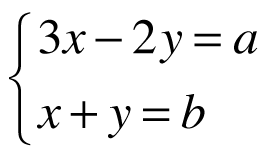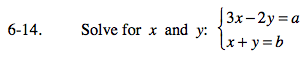### Home > PC > Chapter 6 > Lesson 6.1.1 > Problem6-14

6-14.

Solve for x and y:Homework Help ✎Use either method below.

Method 1: Solve for x in the second equation and substitute into the first equation.

Method 2: Multiply the second equation by 2 and add to the first equation, eliminating y.